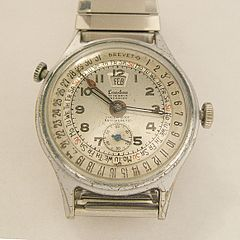# What are the 7 SI Base Units?

Elizabeth Schap, Damien Howard
• Author
Elizabeth Schap

Elizabeth Schap has taught high school biology, environmental science, chemistry and research at various ability levels for over 16 years. She has a Master's in Secondary Science Education from Towson University and a BA with a double concentration in Biology and Communication Arts from Notre Dame of Maryland University. She also has written lesson plans for Scholastic Inc and curricula for National Aquarium in Baltimore.

• Instructor
Damien Howard

Damien has a master's degree in physics and has taught physics lab to college students.

What are SI units? Learn what is a derived unit and study the SI base units and SI derived units. Find out what the fundamental SI units, what the base unit for mass is and examples of SI units. Updated: 08/02/2021

Show

## What are SI Units?

When measuring entities in science and math, universal units are used so all measurements are equal to each other. This allows for ease of comparison and collaboration in the fields of study where people do not physically work together. The International System of Units, or SI units was developed so measurements could remain standard and comparable, yet allow for expansion as new information was discovered and learned. The system has seven fundamental units called base units.

A base unit is the agreed-upon starting unit for each entity, like a second or a meter, and can be used to create other units but cannot be broken themselves. For example, you can measure time in hours and minutes that are derived from seconds (60 seconds in a minute, 60 minutes in an hour). And while you can have a millisecond, the second is the standard fundamental measurement of time.An error occurred trying to load this video.

Try refreshing the page, or contact customer support.

Coming up next: The Metric System: Units and Conversion

### You're on a roll. Keep up the good work!

Replay
Your next lesson will play in 10 seconds
• 0:00 SI Units
• 1:23 Base Units
• 4:12 Derived Units
• 6:14 Lesson Summary
Save Save

Want to watch this again later?

Timeline
Autoplay
Autoplay
Speed Speed## 7 Base SI Units

There are seven base units. Each is used to measure a specific entity and has a symbol used in equations and scientific writing. The symbols (in parenthesis below) allow scientists to work in shorthand and still understand calculations completed by other scientists.

* ampere (A) the rate of electrical flow, or electric current

* candela (cd) luminous intensity, or light intensity, allowing numerical comparisons between lights of different origins (ex: LED light, light bulb, candle)

* kelvin (K) measurement of temperature which has no negative numbers.

* kilogram (kg) mass of an object

* meter (m) distance

* mole (mol) the amount of a substance, used in chemistry, allows scientists to determine the amount of elements in a substance (the amount of oxygen to hydrogen in water).

NOTE: A mole is equal to 6.023 x 10^23 of the material. This is known as Avogadro's Number

* second (s) base unit for time

## Dimensions and SI Units

SI units are helpful in measuring the dimensions of entities. Dimensions help to determine the type of measurement unit a scientist will use when recording data or completing calculations. Length will determine meters are used for measurement, while time needs to be measured in seconds.

### Base Unit for Mass

The concept of mass is often difficult to understand. Mass is the amount of an entity when put on a balance. It is the amount of the entity that is present. It is measured in kilograms as the base unit.

## What is a Derived Unit?

A derived unit is a measurement that can be created by combining multiple base units in an algebraic equation. These units allow scientists to measure other parts of an entity. For example, a scientist can measure the length of a fish tank in meters, but that will not tell the scientist how much water the tank will hold. The amount of water a tank can hold is an example of a derived unit: volume.

Five common derived units are:

• area (m2) meter x meter, measure of a surface
• acceleration (m/s2) meter per second squared, measure of increased speed
• concentration (mol/m3) mole per cubic meter, measure of a solute in a solution
• density (kg/m3) kilogram per cubic meter, measure of compactness
• volume (m3) cubic meter, measure of space a 3D entity holds

To unlock this lesson you must be a Study.com Member.

#### What are examples of derived units?

Some examples of derived units are density, concentration, and volume. Since derived units are calculated buy combining multiple SI units using algebraic equations there are many more.

#### What are base and derived units?

Base units and derived units are both part of the SI measurement system. There are seven base units, ampere, candela, Kelvin, kilogram, meter, mole and second. These are the basic units of measurement for each of their respective entities. Derived units, like mass and volume, are created by combining base units with algebraic formulas.

#### What are SI units?

SI units, or the International System of Units, are the standard scientific units of measurement used by scientists throughout the world. They include both base units and derived units and are an extension of the metric system.

### Register to view this lesson

Are you a student or a teacher?